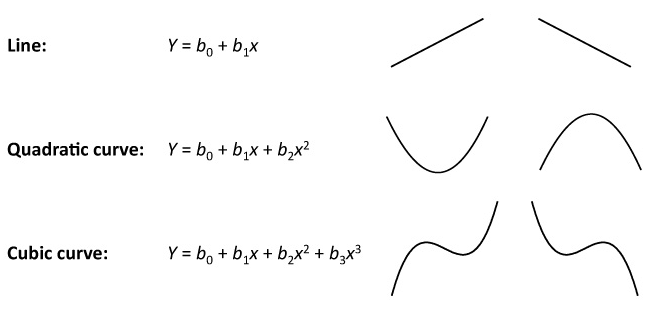4.4

# Interpreting the slope of a trend lineThis is a scatter plot of heights versus ages for about 460 school students.

In iNZight, first adding a linear trend to the plot and then clicking Get Summary shows…

Linear Trend: ${\qquad\qquad}$ height = 5.8 ${*}$ age ${+}$ 85.7

You may remember from school mathematics, that the equation for a straight line is…

…where ${m}$ is the number for the gradient (or slope) of the line, and ${c}$ is the intercept, the ${y}$-value when ${x}$=0.

In our example, ${y}$ is height, ${x}$ is age and Get Summary gives you the values of ${m}$ and ${c}$ that produce the trend line on the graph. We won’t worry about the intercept because we have no data near ${x}$=age=0 and wouldn’t expect this trend relationship to still hold that far away from our data anyway so, for this problem, the intercept is not a meaningful number.

The slope of the line is 5.8. What does this number mean?

The slope of a line is the change in ${y}$ produced by a 1-unit increase in ${x}$.

For our example, the trend line would predict that if someone was 1-year older (${x}$ increases by 1), then they would be about 5.8cm taller (${y}$ increases by 5.8).

Below are similar, but more complicated formulae for curves written in the way typically used by statisticians. Interpretation of the terms for quadratic and cubic curves is much more difficult than for lines and is beyond this course.In each of these formulae, b0 is the intercept. The notation allows more and more powers of ${x}$ to be added in an obvious progression.